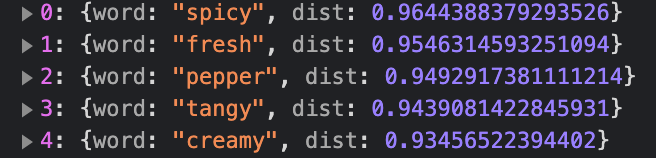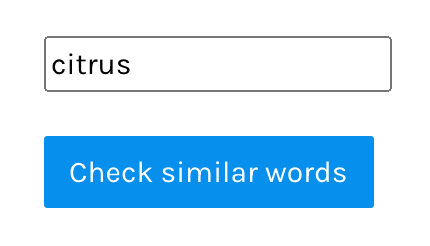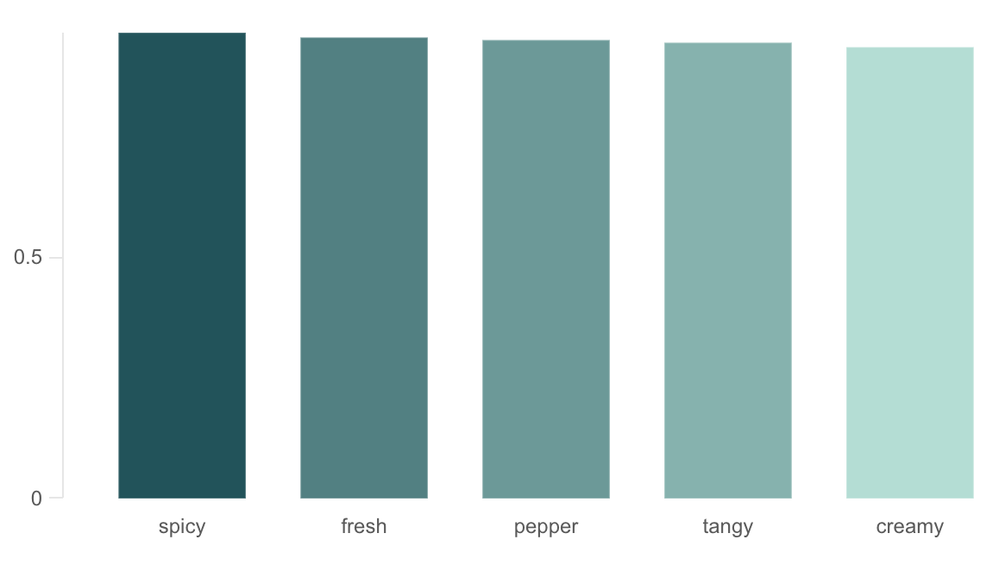# Qlik Design Blog

All about product and Qlik solutions: scripting, data modeling, visual design, extensions, best practices, etc.

Announcements
Support Case Portal has moved to Qlik Community! Read the FAQs to start exploring Support resources.Employee

## Building a Visual Text Analytics app using Qlik and Machine Learning techniques in NodeJS - Part 2

Welcome to the 2nd part of developing a Visual Text Analytics app using Qlik’s open-sourced solutions and a Word embedding technique(Word2Vec). In our previous tutorial , we designed a simple architecture(seen below) for the application that we will learn to develop today.Now, let us try to understand the need for each of these components and their role in our app.

• Front-end : This is the UI of the app that will help the user interact and derive insights.

• Back-end : Consists of 2 sub-components.

• Client-side - This is where we have the Qlik’s visualization libraries Nebula and Picasso.js.

• Server-side - develop the APIs here.

#### CLIENT-SIDE:

So, why do we use two charting libraries from Qlik? Let’s break it down.

For me, when I develop a full-stack solution, I think one of the things that I look into is how to build things quickly and efficiently. Since I have a lot of other components to develop or work with, I want to make sure I don’t end up devoting a significant amount of time to building things from scratch. Nebula.js helps me in this case. It allows me to quickly embed a chart that has already been developed in a Qlik Sense app and use it in my way. All I have to do is to render it in my Visual Analytics app with something like this -

```nuked.render({

element: document.querySelector(".object"),

id: "XHRqzeG"

})```

The second visualization library that I leverage here is  Picasso.js. Picasso enables me to build custom, interactive, component-based visualizations. One of the things that I was looking for with this specific solution was to process textual data, specifically do the word embeddings and return the result of the word embedding to a chart so it helps me in presenting the data visually (note that we are developing a Visual Analytics app).

This is where Picasso.js fits in. It has a similar way of working as D3.js and allows me to work with 2D matrix and array of Objects. I can also use the data as I want in various components of the chart, making it very flexible. Here’s a snippet of how I used my transformed data in a Bar chart.```.then(response => response.json())

.then(data => {

var js_data = [data];

picasso.chart({

element: document.querySelector(".container"),

data: [{

type: "matrix",

data: data

}]

})

});```

Great! So, the gist is -

• Nebula.js - embed already developed Qlik Sense charts (it is quick and easy). Also allows for selections & Qlik specific features.

• Picasso.js - develop a customized chart (use data as we would like to build various chart components)

#### SERVER-SIDE:

The major chunk of our backend is the Server-side component where we develop our APIs. We use the Express.js framework here that helps us to manage routes, requests, etc.

What specific APIs do we have in this app?

• /wordembed : This is the API to perform word embedding using Word2Vec. In this case, we take advantage of the NPM package(https://www.npmjs.com/package/word2vec) which provides a Node.js interface to Google’s Word2Vec implementation. We will be sending the results of the embedding to a Picasso Bar chart.

• /data : Read data processed from Python’s implementation of Principal Component Analysis(PCA) and send it back to a Picasso Scatter plot to visualize the principal components.

Alright! So, we have everything that we need component-wise. Now, let’s quickly understand two things and their need in this solution -

1. Word Embedding - Word2Vec

2. Principal Component Analysis(PCA)

This is where the Machine Learning part comes to play and is key to developing a Visual Text Analytics app like this one.

Since this tutorial is not focused on the implementation of word embeddings/Word2Vec but rather touches upon it from more of an application perspective, we will not delve into details. Simply put, word embedding captures the essence of a word, i.e. their meanings, context, and semantic relationships and converts them into numerical representation (a vector).

For e.g. the word ‘sativa’ can be represented by something like this :

sativa -0.441052 -0.247968 0.463302 0.086262. Please note that the vectors are generally very high-dimensional (in our case we have 300 dimensions).

So, how do we get these vectors?

To derive the vectors, we use the word2vec function like below where

```const w2v = require("word2vec");
w2v.word2vec("cleared_word_embedding.txt", "vectors.txt",
{ size: 300 }, () => {
console.log("generated");
}
);```

These vector representations can then be applied to some interesting use-cases. One of the key tasks that we do by using the vectors in this project is to calculate similarities between words (commonly calculated using  cosine_similarity). So, in the front-end, we allow users to input any word of their choice and they will be visually presented with a chart representing the most similar words. Something like this:This is extremely beneficial for performing text-based analysis. For example, if a user searches for the word ‘citrus’, our Visual Analytics app will present something like this:Here we can see that the context of the word is maintained by the word embedding model and the user is returned with the top 5 most similar words(in descending order) - which are flavors again. If the user wants to then continue their analysis with any other flavor, they can start with the relevant & similar ones. Our API looks like below:

```app.post("/wordembed", (req, res)=>{

var val = req.body.hi

const w2v = require("word2vec");

var sim = model.mostSimilar(val, 5)

res.send(sim);

});

})```

The second part is the Principal Component Analysis(PCA) part. PCA is a technique used to reduce the dimensionality of a high-dimensional dataset (like text, images). As high-dimensional data is very difficult to analyze and visualize, an ideal choice would be to reduce the dimensions by preserving as much information as possible.

Right, but why do we use it in this project?

I wanted to allow users to visualize the words in our vocabulary in 2-dimension so they can explore similarities between them effectively. The best way was to present this information in a Scatter plot. For this specific project, I used the  sklearn’s python implementation of PCA and imported the coordinates in my /data API like below:

```app.get("/data",(req, res)=>{

const path = require('path');
const csv = require('fast-csv');
const data = []
.on('error', (error) => console.error(error))
.on('data', (row) =>
data.push(row)
)
.on('end', () => {
res.send(data);
})

})```

Here is the visualization for the PCA projection.As you can see, words such as ‘depression’, ‘appetite’, ‘relief’ are in close proximity since they are similar. Logically that makes sense as well since these are a couple of things that can be treated using the strains.

Here is the application in action:This brings us to the end of this tutorial on developing a Visual Text Analytics app using Qlik’s open-sourced solutions and Machine Learning techniques such as Word embeddings.

Want to get started building such an app? Here is a  Glitch for developers to remix.